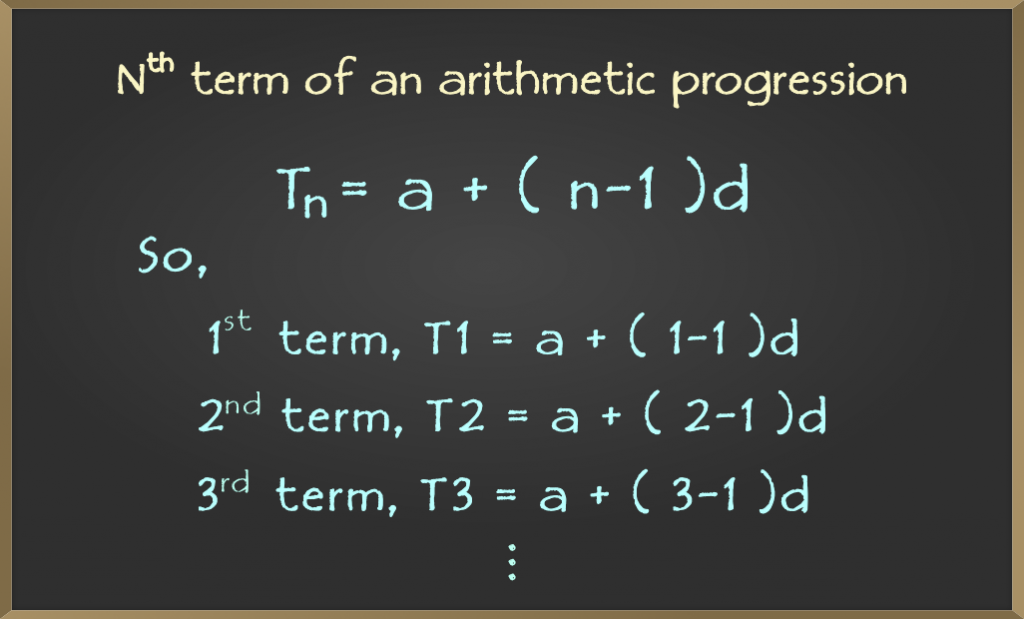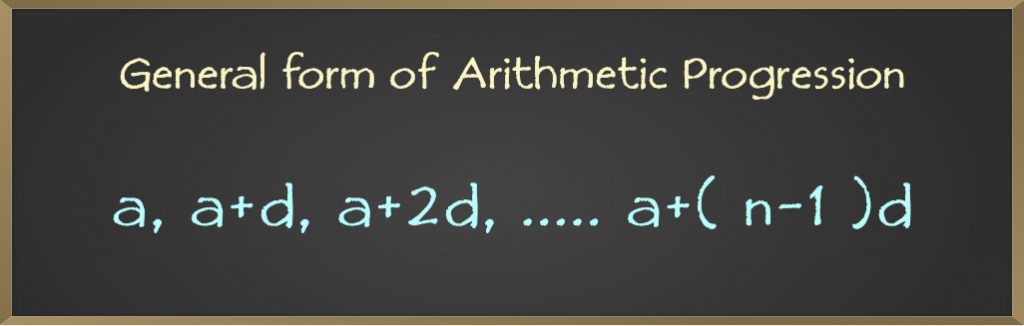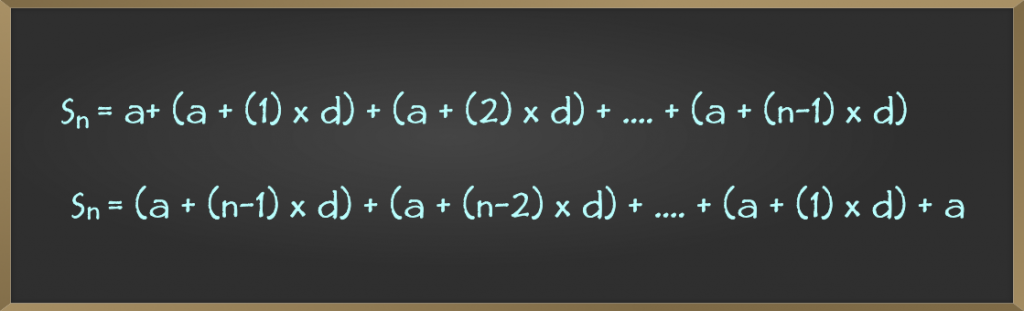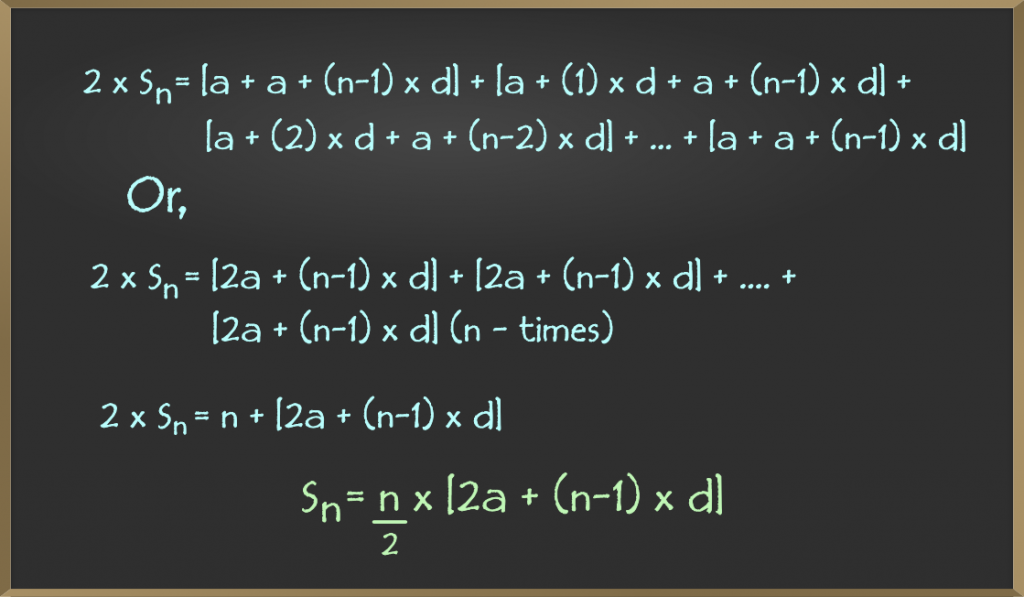Open in App
Not now

# Arithmetic Progression – Sum of First n Terms | Class 10 Maths

• Difficulty Level : Easy
• Last Updated : 15 Sep, 2022

In Algebra a term is either a single number or variable or numbers and variables multiplied together. Terms are separated by + or − signs, or sometimes by the divide.
Examples: In expression 5x – 10 = 1, 5x, 10 and 1 are the terms.

## What is an Arithmetic Progression?

An Arithmetic Progression (AP) or arithmetic series is a sequence of numbers such that the difference between any two consecutive numbers (or terms) is a constant value.
Examples:

```Sequence 1 :  1, 2, 3, 4, 5, 6, ...
Above sequence forms an AP since the difference between any two
consecutive terms is same, which is equal to 1. See below:-
2 - 1 = 1   (2nd - 1st)
3 - 2 = 1   (3rd - 2nd)
...
6 - 5 = 1   (6th - 5th)

Sequence 2 : -4, -2, 0, 2, 4, 6, ...
Similarly above sequence also forms an AP:-
(-2) - (-4) = 2  (2nd - 1st)
(0) - (-2)  = 2  (3rd - 2nd)
...
6 - 4 = 2  (6th - 5th)

Sequence 3 : 1, 3, 5, 9, 4, 10, ...
Above sequence doesn't form an AP as difference between any two
consecutive terms is not same:-
3 - 1 = 2
5 - 3 = 2
9 - 5 = 4
4 - 9 = -5
10 - 4 = 6```

## Finding the formula for Arithmetic Progression

From the examples given above, can you spot the pattern? How can we find the nth term for any provided n?
Let’s try to find out the pattern. Look again at sequence 2:-

```Sequence 2: -4, -2, 0, 2, 4, 6, ...
We see difference as (-2) - (-4) = 2 = ...= 6 - 4 = 2. So difference (say) d = 2.
1st term (say) a = -4```

We can write all the terms as:

1st: -4 + (0) × 2

2nd: -4 + (1) × 2

3rd: -4 + (2) × 2

4th: -4 + (3) × 2

….

….

nth: -4 + (n – 1) × 2

So we see if a is the first term and d is the difference then any nth term, say Tn can be calculated as:Let’s now calculate the 100th term, so for n = 100, we have:

```Tn = a + (n - 1) × d
T100 = (-4) + (100 - 1) × 2
T100 = (-4) + (99) × 2
T100 = 194```

So an AP is of the form:## Applying AP to Divisibility

Arithmetic Progression helps in making mathematical computations easy. Consider the following question:

Question: How many numbers are divisible by 3 in the range of 20 to 100?

Solution: We can use our concept of AP here to solve the problem as follows:

Step 1: Calculate the first number divisible by 3 in the given range:

```20 = 3 × 6 + 2
a = 20 + (3 - remainder)
a = 20 + (3 - 2)
a = 21```

Step 2: Calculate the Last number (nth) in the given range:-

```100 = 3 × 33 + 1
Tn = 100 - remainder
Tn = 100 - 1
Tn = 99```

So we have now an AP whose first term is a and the last term is Tn as follows:
21, 24, 27, …, 99

Now we need to find the number of terms in the above AP, so we use our formula for the nth term for an AP:

```Tn = a + (n - 1) × d
a = 21, Tn = 99, d = 3

99 = 21 + (n - 1) 3
n = 27```

Hence, there are 27 numbers between 20 and 100 that are divisible by 3.

## Solving a Sequence word problem using AP: growth pattern

These types of problems consist of finding a rule (AP formula) for the sequence given in the problem. These are fairly easy.
Consider the following problem:

```Problem: What equation describe the growth pattern of following sequence of numbers:
1, 5, 9, 13, ....
Also calculate 41th term?```

Solution: Let’s see if the sequence follows an AP if any and try to find out the first term and difference:

```5 - 1 = 4
9 - 5 = 4
13 - 9 = 4```

So the difference between any two consecutive terms is the same (equal to 4) so it forms an AP:

`Tn = 1 + (n - 1) × 4`

So the growth pattern is 1 + (n – 1) × 4 and the 41st term is :

```T41 = 1 + (41 - 1) × 4
T41 = 161```

## How to Compute the Sum of first n terms?

Given n and an AP, how do we calculate the sum of the first n terms? Let’s derive the formula for it:
Let Sn denote the sum for the first n terms, we can write Sn in two ways:You see both are the same but written in opposite directions, i.e. in the first elements are from the first term to the last term, and in the second the elements are from last to first.### Computing Sum of first n natural numbers

Problem: Calculate the sum of natural numbers from 1 to 100.

According to anecdotes, when Gauss was in primary school, he was punished by his teacher due to misbehavior.  Gauss was told to add the numbers from 1 to 100 (the teacher gave that considering that a tedious job). But he was able to compute its sum, which is 5050, in a matter of seconds.  How did he do?
He did something like: 1 + 2 + 3 + . . . . + 49 + 50 + (100 – 49) + . . . . + (100 – 2) + (100 – 1) + 100
Which cancels out to 49 * 100 + 100 + 50 = 50 * 100 + 50 = 50(100 + 1). Gauss was great, but we aren’t less though, since we have our formula, we will use that.

Solution: We see the natural numbers (1, 2, 3, …) form an AP and hence we can calculate the sum of the first n terms as follows:
First-term, a = 1
Difference, d = 1
So,

```Sn = (n/2) × [2a + (n - 1) × d]
Sn = (n/2) × [2 × 1 + (n - 1) × 1]
Sn = (n/2) × (n + 1) ```

For n = 100, we have:

```S100 = (100/2) × (100 + 1)
S100 = 5050```

Now you are already good at Arithmetic Progressions!

My Personal Notes arrow_drop_up
Related Articles